Theory and Modern Applications

# Some properties of the Mittag-Leffler functions and their relation with the Wright functions

## Abstract

This paper is a short description of our recent results on an important class of the so-called Mittag-Leffler functions, which became important as solutions of fractional order differential and integral equations, control systems and refined mathematical models of various physical, chemical, economical, management and bioengineering phenomena. We have studied the Mittag-Leffler functions as their typical representatives, including many interesting special cases that have already proven their usefulness in fractional calculus and its applications. We obtained a number of useful relationships between the Mittag-Leffler functions and the Wright functions. The Wright function plays an important role in the solution of a linear partial differential equation. The Wright function, which we denote by $W\left(z;\alpha ,\beta \right)$, is so named in honor of Wright who introduced and investigated this function in a series of notes starting from 1933 in the framework of the asymptotic theory of partitions.

MSC:33E12.

## 1 Introduction

### 1.1 The Mittag-Leffler function

The Mittag-Leffler function is an important function that finds widespread use in the world of fractional calculus. Just as the exponential naturally arises out of the solution to integer order differential equations, the Mittag-Leffler function plays an analogous role in the solution of non-integer order differential equations. In fact, the exponential function itself is a very special form, one of an infinite set of these seemingly ubiquitous functions. The standard definition of Mittag-Leffler  is given as follows:

${E}_{\alpha }\left(z\right)=\sum _{k=0}^{\mathrm{\infty }}\frac{{z}^{k}}{\mathrm{\Gamma }\left(\alpha k+1\right)},\phantom{\rule{1em}{0ex}}\alpha \in C,R\left(\alpha \right)>0,z\in C.$
(1)

A two-parameter function of the M-L (Mittag-Leffler) type is defined by the series expansion 

${E}_{\alpha ,\beta }\left(z\right)=\sum _{k=0}^{\mathrm{\infty }}\frac{{z}^{k}}{\mathrm{\Gamma }\left(\alpha k+\beta \right)}\phantom{\rule{1em}{0ex}}\left(\alpha ,\beta \in C,R\left(\alpha \right)>0,R\left(\beta \right)>0,z\in C\right).$
(2)

The M-L function provides a simple generalization of the exponential function because of the substitution of $n!=\mathrm{\Gamma }\left(n+1\right)n!$ with $\left(n\alpha \right)!=\mathrm{\Gamma }\left(n\alpha +1\right)$. Particular cases of (2) recover elementary functions are recovered

${E}_{1,2}=\frac{{e}^{z}-1}{z},\phantom{\rule{2em}{0ex}}{E}_{2,2}\left({z}^{2}\right)=\frac{sinh\left(z\right)}{z},$

and

${E}_{1/2,1}={e}^{{z}^{2}}erfc\left(-z\right),$

where erf (erfc) denotes the (complementary) error function defined as 

$erfc\left(z\right)=\frac{2}{\sqrt{\pi }}{\int }_{z}^{\mathrm{\infty }}{e}^{-{t}^{2}}\phantom{\rule{0.2em}{0ex}}dt.$

By means of the series representation, a generalization of (1) and (2) is introduced by Prabhakar  as

${E}_{\alpha ,\beta }^{\gamma }\left(z\right)=\sum _{k=0}^{\mathrm{\infty }}\frac{{\left(\gamma \right)}_{n}}{\mathrm{\Gamma }\left(\alpha k+\beta \right)}.\frac{{z}^{k}}{k!}\phantom{\rule{1em}{0ex}}\left(\alpha ,\beta ,\gamma \in C,R\left(\alpha \right)>0,R\left(\beta \right),R\left(\gamma \right)>0,z\in C\right),$
(3)

where ${\left(\gamma \right)}_{n}$ is the Pochhammer symbol  given by

${\left(\lambda \right)}_{n}=\frac{\mathrm{\Gamma }\left(\lambda +n\right)}{\mathrm{\Gamma }\left(\lambda \right)}=\left\{\begin{array}{cc}1\hfill & \left(n=0;\lambda \ne 0\right),\hfill \\ {\left(\gamma \right)}_{n}=\gamma \left(\gamma +1\right)\left(\gamma +2\right)\cdots \left(\gamma +n-1\right)\hfill & \left(n\in N;\lambda \in C\right).\hfill \end{array}$

Note that

${E}_{1,1}^{1}\left(z\right)={e}^{z},\phantom{\rule{2em}{0ex}}{E}_{\alpha ,1}^{1}\left(z\right)={E}_{\alpha }\left(z\right),\phantom{\rule{2em}{0ex}}{E}_{\alpha ,\beta }^{1}\left(z\right)={E}_{\alpha ,\beta }\left(z\right).$

Some new properties of the Mittag-Leffler function, including a definite integral and recurrence relation, were investigated in [6, 7].

### 1.2 The Wright function

The Wright function plays an important role in the solution of a linear partial differential equation. The Wright function, which we denote by $W\left(z;\alpha ,\beta \right)$, is so named in honor of Wright, who introduced and investigated this function in a series of notes starting from 1933 in the framework of the asymptotic theory of partitions. This function was introduced that related Mittag-Leffler . We obtained a number of useful relationships between the Mittag-Leffler functions and the Wright functions.

#### 1.2.1 Definition

The Wright function is defined by the series representation, convergent in the whole z-complex plane 

$W\left(z;\alpha ,\beta \right)=\sum _{k=0}^{\mathrm{\infty }}\frac{{z}^{k}}{k!\mathrm{\Gamma }\left(\alpha k+\beta \right)}.$

#### 1.2.2 The integral representation of the Wright function

$W\left(z;\alpha ,\beta \right)=\frac{1}{2\pi i}{\int }_{Ha}exp\left\{u+z{u}^{-\alpha }\right\}{u}^{-\beta }\phantom{\rule{0.2em}{0ex}}du,$

where Ha denotes the Hankel path. To prove the Hankel path, let us write the integrated function in the form of a power series in z and perform term-by-term integration using the integral representation formula for the reciprocal gamma function

$\frac{1}{\mathrm{\Gamma }\left(z\right)}={\int }_{Ha}{e}^{\zeta }{\zeta }^{z}\phantom{\rule{0.2em}{0ex}}d\zeta .$

In fact,

$\begin{array}{rcl}W\left(z;\alpha ,\beta \right)& =& \frac{1}{2\pi i}{\int }_{Ha}exp\left\{u+z{u}^{-\alpha }\right\}{u}^{-\beta }\phantom{\rule{0.2em}{0ex}}du=\frac{1}{2\pi i}{\int }_{Ha}{e}^{u}\left[\sum _{n=0}^{\mathrm{\infty }}\frac{{z}^{n}}{n!}{u}^{-\alpha n}\right]{u}^{-\beta }\phantom{\rule{0.2em}{0ex}}du\\ =& \sum _{n=0}^{\mathrm{\infty }}\frac{{z}^{n}}{n!}\left[\frac{1}{2\pi i}{\int }_{Ha}{e}^{u}{u}^{-\alpha n-\beta }\phantom{\rule{0.2em}{0ex}}du\right]=\sum _{n=0}^{\mathrm{\infty }}\frac{{z}^{n}}{n!\mathrm{\Gamma }\left(\alpha n+\beta \right)}.\end{array}$

#### 1.2.3 The Laplace transform of the Wright function

We recall that the Mittag-Leffler function plays fundamental roles in applications of fractional calculus like fractional relaxation and fractional oscillation . Kiryakova introduced and studied the multi-index Mittag-Leffler functions as their typical representatives, including many interesting special cases that have already proven their usefulness in FC and its applications . Srivatava and Tomovski introduced and investigated the fractional calculus with an integral operator which contains the following family of generalized Mittag-Leffler functions . Haubold, Mathaian and Saxena studied the Mittag-Leffler functions and their applications . There is an interesting link between the Wright function and the Mittag-Leffler function. We now point out that the Wright function is related to the Mittag-Leffler function through the following Laplace transform pair: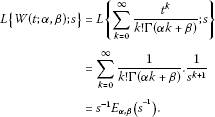## 2 Some properties of the Mittag-Leffler functions

Theorem 1 (Derivative of the Mittag-Leffler function)

If $\alpha ,\beta ,\gamma \in C$, $R\left(\alpha \right)>0$, $R\left(\beta \right)>0$, $R\left(\gamma \right)>0$, $z\in C$ and $r,n\in N$, then

$\frac{{d}^{n}}{d{x}^{n}}\left[{z}^{\beta -1}{E}_{\alpha ,\beta +r\alpha }^{\gamma }\left(z\right)\right]={z}^{\beta -n-1}{E}_{\alpha ,\beta +r\alpha -n}^{\gamma }\left(z\right).$

Proof Using definition (3), we have that

$\frac{{d}^{n}}{d{x}^{n}}\left[{z}^{\beta -1}{E}_{\alpha ,\beta +r\alpha }^{\gamma }\left(z\right)\right]=\sum _{k=0}^{\mathrm{\infty }}\frac{{\left(\gamma \right)}_{k}}{\mathrm{\Gamma }\left(\alpha \left(k+r\right)+\beta -n\right)}\frac{{z}^{k+\beta -1-n}}{k!}={z}^{\beta -n-1}{E}_{\alpha ,\beta +r\alpha -n}^{\gamma }\left(z\right).$

The Wright function is expressed with help of the Mittag-Leffler function:

$\frac{{d}^{n}}{d{x}^{n}}\left[{z}^{\beta -1}{E}_{\alpha ,\beta +r\alpha }^{\gamma }\left(z\right)\right]=\sum _{k=0}^{\mathrm{\infty }}{z}^{\beta -1-n}{\left(\gamma \right)}_{k}W\left(z;\alpha ,\beta +r\alpha -n\right).$

□

Theorem 2 (Integration of the Mittag-Leffler function)

If $\alpha ,\beta ,\gamma \in C$, $R\left(\alpha \right)>0$, $R\left(\beta \right)>0$, $R\left(\gamma \right)>0$, $z\in C$ and $r\in N$, then

${\int }_{0}^{z}{z}^{\beta +r\alpha -1}{E}_{\alpha ,\beta +r\alpha }^{\gamma }\left(z\right)\phantom{\rule{0.2em}{0ex}}dz={z}^{\beta +r\alpha }{E}_{\alpha ,\beta +r\alpha +1}^{\gamma }\left(z\right).$

Proof According to the Mittag-Leffler function, we have

${z}^{\beta +r\alpha -1}{E}_{\alpha ,\beta +r\alpha }^{\gamma }\left(z\right)=\sum _{k=0}^{\mathrm{\infty }}\frac{{\left(\gamma \right)}_{k}}{\mathrm{\Gamma }\left(\alpha \left(k+r\right)+\beta \right)}.\frac{{z}^{k+r\alpha +\beta -1}}{k!}.$

Integrating both sides gives

$\begin{array}{rcl}{\int }_{0}^{z}{z}^{\beta +r\alpha -1}{E}_{\alpha ,\beta +r\alpha }^{\gamma }\left(z\right)\phantom{\rule{0.2em}{0ex}}dz& =& {\int }_{0}^{z}\sum _{k=0}^{\mathrm{\infty }}\frac{{\left(\gamma \right)}_{k}}{\mathrm{\Gamma }\left(\alpha \left(k+r\right)+\beta \right)}.\frac{{z}^{k+r\alpha +\beta -1}}{k!}\phantom{\rule{0.2em}{0ex}}dz\\ =& \sum _{k=0}^{\mathrm{\infty }}\frac{{\left(\gamma \right)}_{k}}{\mathrm{\Gamma }\left(\alpha \left(k+r\right)+\beta +1\right)}.\frac{{z}^{k+r\alpha +\beta }}{k!}\\ =& {z}^{\beta +r\alpha }{E}_{\alpha ,\beta +r\alpha +1}^{\gamma }\left(z\right).\end{array}$

Relation with the Wright functions is as follows:

$\begin{array}{rcl}{\int }_{0}^{z}{z}^{\beta +r\alpha -1}{E}_{\alpha ,\beta +r\alpha }^{\gamma }\left(z\right)\phantom{\rule{0.2em}{0ex}}dz& =& {\int }_{0}^{z}\sum _{k=0}^{\mathrm{\infty }}\frac{{\left(\gamma \right)}_{k}}{\mathrm{\Gamma }\left(\alpha \left(k+r\right)+\beta \right)}.\frac{{z}^{k+r\alpha +\beta -1}}{k!}\phantom{\rule{0.2em}{0ex}}dz\\ =& \sum _{k=0}^{\mathrm{\infty }}{z}^{\beta +r\alpha }{\left(\gamma \right)}_{k}W\left(z;\alpha ,\beta +r\alpha +1\right).\end{array}$

□

Theorem 3 Let $\alpha ,\beta ,\gamma \in C$, $R\left(\alpha \right)>0$, $R\left(\beta \right)>0$, $R\left(\gamma \right)>0$, $z\in C$, $r\in N$, then

${E}_{\alpha ,\beta }^{\gamma }\left(z\right)=\beta {E}_{\alpha ,\beta +1}^{\gamma }\left(z\right)+\alpha z\frac{d}{dz}{E}_{\alpha ,\beta +1}^{\gamma }\left(z\right).$

Proof By definition (3), we have that

$\begin{array}{rcl}\beta {E}_{\alpha ,\beta +1}^{\gamma }\left(z\right)+\alpha z\frac{d}{dz}{E}_{\alpha ,\beta +1}^{\gamma }\left(z\right)& =& \beta {E}_{\alpha ,\beta +1}^{\gamma }\left(z\right)+\alpha z\frac{d}{dz}\sum _{n=0}^{\mathrm{\infty }}\frac{{\left(\gamma \right)}_{n}}{\mathrm{\Gamma }\left(\alpha n+\beta +1\right)}.\frac{{z}^{n}}{n!}\\ =& \sum _{n=0}^{\mathrm{\infty }}\frac{\beta {\left(\gamma \right)}_{n}}{\mathrm{\Gamma }\left(\alpha n+\beta +1\right)}.\frac{{z}^{n}}{n!}+\sum _{n=0}^{\mathrm{\infty }}\frac{\alpha n{\left(\gamma \right)}_{n}}{\mathrm{\Gamma }\left(\alpha n+\beta +1\right)}.\frac{{z}^{n}}{n!}\\ =& {E}_{\alpha ,\beta }^{\gamma }\left(z\right).\end{array}$

Relation with the Wright functions is as follows:

$\begin{array}{rcl}\beta {E}_{\alpha ,\beta +1}^{\gamma }\left(z\right)+\alpha z\frac{d}{dz}{E}_{\alpha ,\beta +1}^{\gamma }\left(z\right)& =& \beta {E}_{\alpha ,\beta +1}^{\gamma }\left(z\right)+\alpha z\frac{d}{dz}\sum _{n=0}^{\mathrm{\infty }}\frac{{\left(\gamma \right)}_{n}}{\mathrm{\Gamma }\left(\alpha n+\beta +1\right)}.\frac{{z}^{n}}{n!}\\ =& \sum _{n=0}^{\mathrm{\infty }}\frac{\beta {\left(\gamma \right)}_{n}}{\mathrm{\Gamma }\left(\alpha n+\beta +1\right)}.\frac{{z}^{n}}{n!}+\sum _{n=0}^{\mathrm{\infty }}\frac{\alpha n{\left(\gamma \right)}_{n}}{\mathrm{\Gamma }\left(\alpha n+\beta +1\right)}.\frac{{z}^{n}}{n!}\\ =& \sum _{n=0}^{\mathrm{\infty }}{\left(\gamma \right)}_{n}W\left(z;\alpha ,\beta \right).\end{array}$

□

Theorem 4 For $\alpha ,\beta ,\gamma \in C$, $R\left(\alpha \right)>0$, $R\left(\beta \right)>0$, $R\left(\gamma \right)>0$, $z\in C$, $r\in N$. ‘Note that’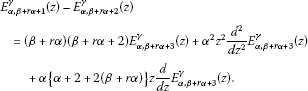(4)

Proof We have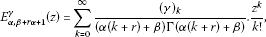(5)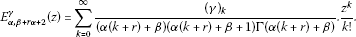(6)

Equation (6) can be written as follows:

$\begin{array}{rcl}{E}_{\alpha ,\beta +r\alpha +2}^{\gamma }\left(z\right)& =& \sum _{k=0}^{\mathrm{\infty }}\left[\frac{1}{\alpha \left(k+r\right)+\beta }-\frac{1}{\alpha \left(k+r\right)+\beta +1}\right]\frac{{z}^{k}{\left(\gamma \right)}_{k}}{\mathrm{\Gamma }\left(\alpha \left(k+r\right)+\beta \right)k!}\\ =& {E}_{\alpha ,\beta +r\alpha +1}^{\gamma }\left(z\right)-\sum _{k=0}^{\mathrm{\infty }}\frac{{z}^{k}{\left(\gamma \right)}_{k}}{\left(\alpha \left(k+r\right)+\beta +1\right)\mathrm{\Gamma }\left(\alpha \left(k+r\right)+\beta \right)k!}.\end{array}$
(7)

We find from equation (7) that

$\begin{array}{rcl}{E}_{\alpha ,\beta +r\alpha +1}^{\gamma }\left(z\right)-{E}_{\alpha ,\beta +r\alpha +2}^{\gamma }\left(z\right)& =& \sum _{k=0}^{\mathrm{\infty }}\frac{{z}^{k}{\left(\gamma \right)}_{k}}{\left(\alpha \left(k+r\right)+\beta +1\right)\mathrm{\Gamma }\left(\alpha \left(k+r\right)+\beta \right)k!}\\ =& \frac{{z}^{k}{\left(\gamma \right)}_{k}}{k!}\left(\frac{1}{\left(\alpha \left(k+r\right)+\beta +2\right)\left(\alpha \left(k+r\right)+\beta +1\right)\mathrm{\Gamma }\left(\alpha \left(k+r\right)+\beta \right)}\\ +\frac{1}{\left(\alpha \left(k+r\right)+\beta +2\right)\mathrm{\Gamma }\left(\alpha \left(k+r\right)+\beta \right)}\right)\end{array}$
(8)

or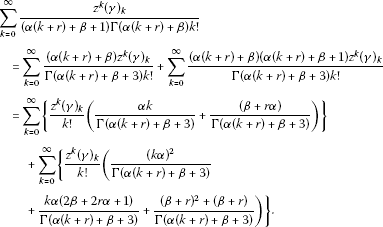(9)

We now say each summation on the right-hand side of equation (9) is as follows:

$\begin{array}{rcl}\frac{{d}^{2}}{d{z}^{2}}\left\{{z}^{2}{E}_{\alpha ,\beta +r\alpha +3}^{\gamma }\left(z\right)\right\}& =& \sum _{k=0}^{\mathrm{\infty }}\frac{\left(k+2\right)\left(k+1\right){\left(\gamma \right)}_{k}}{\mathrm{\Gamma }\left(\alpha \left(k+r\right)+\beta +3\right)}.\frac{{z}^{k}}{k!}\\ =& \sum _{k=0}^{\mathrm{\infty }}\frac{{k}^{2}{\left(\gamma \right)}_{k}}{\mathrm{\Gamma }\left(\alpha \left(k+r\right)+\beta +3\right)}.\frac{{z}^{k}}{k!}\\ +3\sum _{k=0}^{\mathrm{\infty }}\frac{k{\left(\gamma \right)}_{k}}{\mathrm{\Gamma }\left(\alpha \left(k+r\right)+\beta +3\right)}.\frac{{z}^{k}}{k!}+2{E}_{\alpha ,\beta +r\alpha +3}^{\gamma }\left(z\right)\end{array}$
(10)

or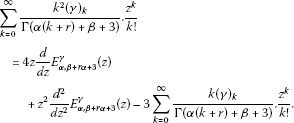(11)

We find from equation (11) that

$\sum _{k=0}^{\mathrm{\infty }}\frac{{k}^{2}{\left(\gamma \right)}_{k}}{\mathrm{\Gamma }\left(\alpha \left(k+r\right)+\beta +3\right)}.\frac{{z}^{k}}{k!}=z\frac{d}{dz}{E}_{\alpha ,\beta +r\alpha +3}^{\gamma }\left(z\right)+{z}^{2}\frac{{d}^{2}}{d{z}^{2}}{E}_{\alpha ,\beta +r\alpha +3}^{\gamma }\left(z\right).$
(12)

Using equations (9), (11), and (12), we get

$\begin{array}{c}{E}_{\alpha ,\beta +r\alpha +1}^{\gamma }\left(z\right)-{E}_{\alpha ,\beta +r\alpha +2}^{\gamma }\left(z\right)\hfill \\ \phantom{\rule{1em}{0ex}}=\left(\beta +r\alpha \right)\left(\beta +r\alpha +2\right){E}_{\alpha ,\beta +r\alpha +3}^{\gamma }\left(z\right)+{\alpha }^{2}{z}^{2}\frac{{d}^{2}}{d{z}^{2}}{E}_{\alpha ,\beta +r\alpha +3}^{\gamma }\left(z\right)\hfill \\ \phantom{\rule{2em}{0ex}}+\alpha \left\{\alpha +2+2\left(\beta +r\alpha \right)\right\}z\frac{d}{dz}{E}_{\alpha ,\beta +r\alpha +3}^{\gamma }\left(z\right).\hfill \end{array}$

Relation with the Wright functions is as follows:

$\begin{array}{c}{E}_{\alpha ,\beta +r\alpha +1}^{\gamma }\left(z\right)-{E}_{\alpha ,\beta +r\alpha +2}^{\gamma }\left(z\right)\hfill \\ \phantom{\rule{1em}{0ex}}=\sum _{k=0}^{\mathrm{\infty }}\left(\beta +r\alpha \right)\left(\beta +r\alpha +2\right){\left(\gamma \right)}_{k}W\left(z;\alpha ,\beta +r\alpha +3\right)\hfill \\ \phantom{\rule{2em}{0ex}}+\sum _{k=0}^{\mathrm{\infty }}{\alpha }^{2}{z}^{2}{\left(\gamma \right)}_{k}\frac{{d}^{2}}{d{z}^{2}}W\left(z;\alpha ,\beta +r\alpha +3\right)\hfill \\ \phantom{\rule{2em}{0ex}}+\sum _{k=0}^{\mathrm{\infty }}\alpha \left\{\alpha +2+2\left(\beta +r\alpha \right)\right\}{\left(\gamma \right)}_{k}z\frac{d}{dz}W\left(z;\alpha ,\beta +r\alpha +3\right).\hfill \end{array}$

□

Theorem 5 If $\alpha ,\beta ,\gamma \in C$, $R\left(\alpha \right)>0$, $R\left(\beta \right)>0$, $R\left(\gamma \right)>0$, $z\in C$, $r\in N$, then

${z}^{r}{E}_{\alpha ,\beta +r\alpha }^{\gamma }\left(z\right)={E}_{\alpha ,\beta }^{\gamma }\left(z\right)-\sum _{k=0}^{r-1}\frac{{\left(\gamma \right)}_{k}}{\mathrm{\Gamma }\left(k\alpha +\beta \right)}\frac{{z}^{k}}{k!}.$
(13)

Proof We have from (13)

$\sum _{k=r}^{\mathrm{\infty }}\frac{{\left(\gamma \right)}_{k}}{\mathrm{\Gamma }\left(k\alpha +\beta \right)}\frac{{z}^{k}}{k!}={E}_{\alpha ,\beta }^{\gamma }\left(z\right)-\sum _{k=o}^{r-1}\frac{{\left(\gamma \right)}_{k}}{\mathrm{\Gamma }\left(k\alpha +\beta \right)}\frac{{z}^{k}}{k!}.$

For $r=2,3,4,\dots$ , we obtain

$\begin{array}{c}{z}^{2}{E}_{\alpha ,\beta +2\alpha }^{\gamma }\left(z\right)={E}_{\alpha ,\beta }^{\gamma }\left(z\right)-\frac{1}{\mathrm{\Gamma }\left(\beta \right)}-\frac{{\left(\gamma \right)}_{1}z}{\mathrm{\Gamma }\left(\alpha +\beta \right)},\hfill \\ {z}^{3}{E}_{\alpha ,\beta +3\alpha }^{\gamma }\left(z\right)={E}_{\alpha ,\beta }^{\gamma }\left(z\right)-\frac{1}{\mathrm{\Gamma }\left(\beta \right)}-\frac{{\left(\gamma \right)}_{1}z}{\mathrm{\Gamma }\left(\alpha +\beta \right)}-\frac{{\left(\gamma \right)}_{2}{z}^{2}}{\mathrm{\Gamma }\left(2\alpha +\beta \right)},\hfill \\ {z}^{4}{E}_{\alpha ,\beta +4\alpha }^{\gamma }\left(z\right)={E}_{\alpha ,\beta }^{\gamma }\left(z\right)-\frac{1}{\mathrm{\Gamma }\left(\beta \right)}-\frac{{\left(\gamma \right)}_{1}z}{\mathrm{\Gamma }\left(\alpha +\beta \right)}-\frac{{\left(\gamma \right)}_{2}{z}^{2}}{\mathrm{\Gamma }\left(2\alpha +\beta \right)}-\frac{{\left(\gamma \right)}_{3}{z}^{3}}{\mathrm{\Gamma }\left(3\alpha +\beta \right)},\hfill \\ ⋮\hfill \\ \sum _{k=r}^{\mathrm{\infty }}\frac{{\left(\gamma \right)}_{k}}{\mathrm{\Gamma }\left(k\alpha +\beta \right)}\frac{{z}^{k}}{k!}={E}_{\alpha ,\beta }^{\gamma }\left(z\right)-\sum _{k=o}^{r-1}\frac{{\left(\gamma \right)}_{k}}{\mathrm{\Gamma }\left(k\alpha +\beta \right)}\frac{{z}^{k}}{k!}.\hfill \end{array}$

Relation with the Wright functions is as follows:

$\sum _{k=r}^{\mathrm{\infty }}\frac{{\left(\gamma \right)}_{k}}{\mathrm{\Gamma }\left(k\alpha +\beta \right)}\frac{{z}^{k}}{k!}=\sum _{k=0}^{\mathrm{\infty }}{\left(\gamma \right)}_{k}W\left(z;\alpha ,\beta \right)-\sum _{k=0}^{r-1}{\left(\gamma \right)}_{k}W\left(z;\alpha ,\beta \right).$

□

## References

1. Mittag-Leffler G:Sur la nouvelle fontion ${E}_{\alpha }$. Comptes Rendus Hebdomadaires Des Seances Del Academie Des Sciences, Paris 2 1903, 137: 554–558.

2. Wiman A: Über den fundamental Satz in der Theorie der Funktionen. Acta Math. 1905, 29: 191–201. 10.1007/BF02403202

3. Podlubny I: Fractional Differential Equations. Academic Press, San Diego; 1999.

4. Prabhakar TR: A singular integral equation with a generalized Mittag-Leffler function in the kernel. Yokohama Math. J. 1971, 19: 7–15.

5. Rainville ED: Special Functions. Macmillan, New York; 1960.

6. Gupta IS, Debnath L: Some properties of the Mittag-Leffer functions. Integral Transforms Spec. Funct. 2007, 18(5):329–336. 10.1080/10652460601090216

7. Peng J, Li K: A note on property of the Mittag-Leffler function. J. Math. Anal. Appl. 2010, 370(2):635–638. 10.1016/j.jmaa.2010.04.031

8. Wright EM: On the coefficients of power series having exponential singularities. J. Lond. Math. Soc. 1933, 8: 71–79. 10.1112/jlms/s1-8.1.71

9. Wright EM: The asymptotic expansion of the generalized Bessel function. Proc. Lond. Math. Soc. 1935, 38: 257–270. 10.1112/plms/s2-38.1.257

10. Wright EM: The asymptotic expansion of the generalized hypergeometric function. J. Lond. Math. Soc. 1935, 10: 287–293.

11. Wright EM: The generalized Bessel function of order greater than one. Q. J. Math., Oxford Ser. 1940, 11: 36–48. 10.1093/qmath/os-11.1.36

12. Erdelyi A, Magnus W, Oberhettinger F, Tricomi FG 3. In Higher Transcendental Functions. McGraw-Hill, New York; 1954. chapter 18

13. Mainardi F, Mura A, Pagnini G: The M -Wright function in time-fractional diffusion processes: a tutorial survey. Int. J. Differ. Equ. 2010. doi:10.1155/2010/104505

14. Mainardi F, Gorenflo R: Time-fractional derivatives in relaxation processes: a tutorial survey. Fract. Calc. Appl. Anal. 2007, 10: 269–308.

15. Achar BNN, Hanneken JW, Clarke T: Damping characteristics of a fractional oscillator. Physica A 2004, 339: 311–319. 10.1016/j.physa.2004.03.030

16. Mainardi F: Fractional Calculus and Waves in Linear Viscoelasticity. Imperial College Press, London; 2010.

17. Kiryakova V: The multi-index Mittag-Leffler functions as an important class of special functions of fractional calculus. Comput. Math. Appl. 2010, 59: 1885–1895. 10.1016/j.camwa.2009.08.025

18. Srivastava HM, Tomovski Ž: Fractional calculus with an integral operator containing a generalized Mittag-Leffler function in the kernel. Appl. Math. Comput. 2009, 211: 198–210. 10.1016/j.amc.2009.01.055

19. Haubold HJ, Mathaiand AM, Saxena RK: Mittag-Leffler functions and their applications. J. Appl. Math. 2011., 2011: Article ID 298628. doi:10.1155/2011/298628

## Acknowledgements

This work was supported by the scientific and technological research council of Turkey (TUBITAK).

## Author information

Authors

### Corresponding author

Correspondence to Muhammet Kurulay.

### Competing interests

The authors declare that they have no competing interests.

### Authors’ contributions

MK defined the research theme. MK designed theorems and proofs, analyzed the data, interpreted the results and wrote the paper. MB participated drafted the manuscript. All authors read and approved the final manuscript.

## Rights and permissions

Reprints and Permissions

Kurulay, M., Bayram, M. Some properties of the Mittag-Leffler functions and their relation with the Wright functions. Adv Differ Equ 2012, 181 (2012). https://doi.org/10.1186/1687-1847-2012-181